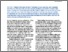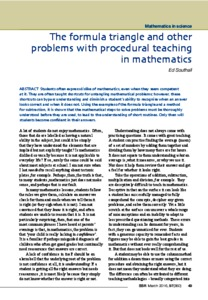Southall, Edward (2016) The formula triangle and other problems with procedural teaching in mathematics. School Science Review, 97 (360). pp. 49-53. ISSN 0036-6811
Abstract

Students often express dislike of mathematics, even when they seem competent
at it. They are often taught shortcuts for untangling mathematical problems; however, these
shortcuts can bypass understanding and diminish a student’s ability to recognise when an answer
looks correct and when it does not. Using the examples of the formula triangle and a method
for subtraction, it is shown that the mathematical steps to solve problems must be thoroughly understood before they are used, to lead to the understanding of short routines. Only then will students become confident in their answers.

Information
Library
DocumentsPreview
SSR March 2016 049-053 Southall.pdf - Published Version